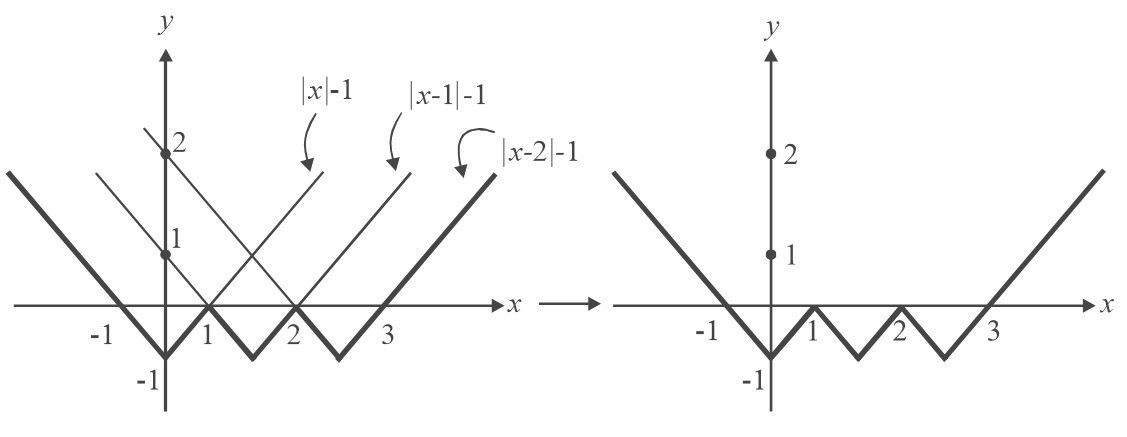# Functions Set 4

Go back to  'SOLVED EXAMPLES'

Example – 6

Plot the curve   $$y = \min \left\{ {|x| - 1,\,\,\,|x - 1| - 1,\,\,\,|x - 2| - 1} \right\}$$ .

Solution: What does such a statement mean? It means that for each value of x , we evaluate all the three quantities on the RHS, select the minimum of the three, and plot that value of y.

What we can do is plot the separate graphs for these three quantities on the same axes and select those portions that lie lowermost, out of all the three.The darker line segments on the left hand side diagram show the minimum value out of all the three, considered at each point x. This is therefore the graph of y.

Example – 7

Solve the equation for x : \begin{align}\frac{1}{{[x]}} + \frac{1}{{[2x]}} = \{ x\} + \frac{1}{3}\end{align}

Solution: A solution is not evident by mere observation. But it can be noted that the RHS is always positive: therefore, the LHS must be positive and hence x > 0.

Also, for the LHS to be defined,  $$x > 1{\rm{\;\;}}and{\rm{\;\;}}2x > 1{\rm{ }} \Rightarrow {\rm{ }}x > 1\;and\;\;hence[x] > 1.$$

Let I be the integral and f the fractional part of x.

$\Rightarrow \frac{1}{I} + \frac{1}{{2I + [2f]}} = f + \frac{1}{3}$

We have retained $$[2f]$$  since 2f could be greater than 1 and hence $$[2f]$$  is not necessarily 0.

We will have to consider two different cases separately:

(i) $$\fbox{{f < \frac{1}{2}}}$$  $$\Rightarrow$$ \begin{align}\frac{1}{I} + \frac{1}{{2I}}\end{align}      =  $$f + \frac{1}{3}$$

{\rm{}} \Rightarrow \begin{align}\frac{3}{{2I}}{\rm{ }} = {\rm{}}f + \frac{1}{3}\end{align}

Now we can substitute different values of I; if these give valid values for f such that $$f < \frac{1}{2}$$ , we accept these solutions.

 $I = 1{\rm{}} \Rightarrow {\rm{ }}f = \frac{7}{6}$ [not acceptable] $I = 2{\rm{ }} \Rightarrow f = \frac{5}{{12}}$ [acceptable] $I = 3{\rm{ }} \Rightarrow {\rm{ }}f = \frac{1}{6}{\rm{}}$ [acceptable] $I = 4{\rm{ }} \Rightarrow {\rm{ }}f = \frac{1}{{24}}$ [acceptable] $I = 5{\rm{ }} \Rightarrow {\rm{ }}f < 0$ [not acceptable]

No more solutions will exist since f  becomes   $$< {\rm{ }}0{\rm{ }}forI > 5$$

(ii) $$\fbox{{f \ge \frac{1}{2}}}$$   $$\Rightarrow$$\begin{align}\frac{1}{I} + \frac{1}{{2I + 1}}\end{align}    = $$f + \frac{1}{3}$$

 $$I = 1{\rm{}} \Rightarrow {\rm{ }}f = 1{\rm{}}$$ [not acceptable] I = 2 \Rightarrow f = \begin{align}\frac{{11}}{{30}}{\rm{ }}(f\;does\;not\;satisfy\;f \ge \frac{1}{2}){\rm{}}\end{align} [not acceptable] I = 3 \Rightarrow {\rm{ }}f =\begin{align} \frac{1}{7}\end{align} [not acceptable] $$I = 4 \Rightarrow f = 1/36$$ [not acceptable] $$I = 5 \Rightarrow f < 0$$ [not acceptable]

Hence the valid solutions are \begin{align}x = I + f = \frac{{29}}{{12}},\,\,\,\frac{{19}}{6},\,\,\,\frac{{97}}{{24}}\end{align}

Learn from the best math teachers and top your exams

• Live one on one classroom and doubt clearing
• Practice worksheets in and after class for conceptual clarity
• Personalized curriculum to keep up with school